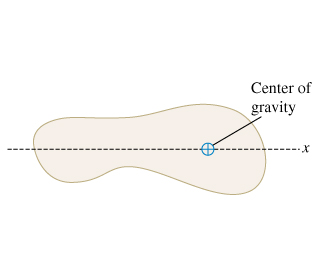# Problem: The center of gravity of an irregular object of mass 5.10 g is shown in the figure.You need to move the center of gravity 2.30 cm to the left by gluing on a tiny 1.60-g mass, which will then be considered as part of the object.Where should you attach this additional mass?

###### FREE Expert Solution

X-coordinate of the center of mass:

$\overline{){{\mathbf{x}}}_{\mathbf{c}\mathbf{m}}{\mathbf{=}}\frac{{\mathbf{m}}_{\mathbf{1}}{\mathbf{x}}_{\mathbf{1}}\mathbf{+}{\mathbf{m}}_{\mathbf{2}}{\mathbf{x}}_{\mathbf{2}}\mathbf{+}\mathbf{.}\mathbf{.}\mathbf{.}\mathbf{+}{\mathbf{m}}_{\mathbf{n}}{\mathbf{x}}_{\mathbf{n}}}{{\mathbf{m}}_{\mathbf{1}}\mathbf{+}{\mathbf{m}}_{\mathbf{2}}\mathbf{+}\mathbf{.}\mathbf{.}\mathbf{.}\mathbf{+}{\mathbf{m}}_{\mathbf{n}}}}$

Let x be the distance between the object mass and the tiny mass.###### Problem Details

The center of gravity of an irregular object of mass 5.10 g is shown in the figure.You need to move the center of gravity 2.30 cm to the left by gluing on a tiny 1.60-g mass, which will then be considered as part of the object.

Where should you attach this additional mass?

Frequently Asked Questions

What scientific concept do you need to know in order to solve this problem?

Our tutors have indicated that to solve this problem you will need to apply the Review: Center of Mass concept. You can view video lessons to learn Review: Center of Mass. Or if you need more Review: Center of Mass practice, you can also practice Review: Center of Mass practice problems.